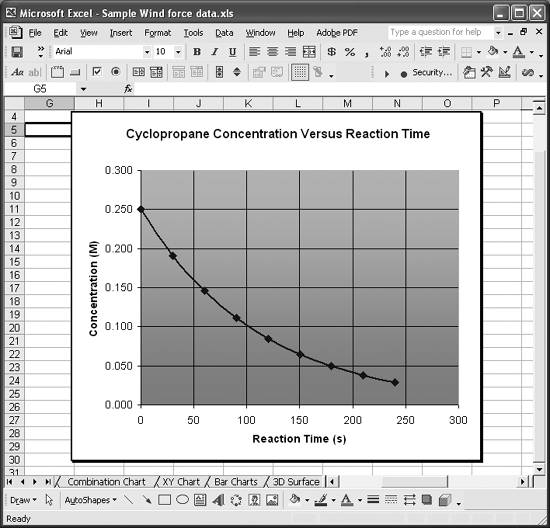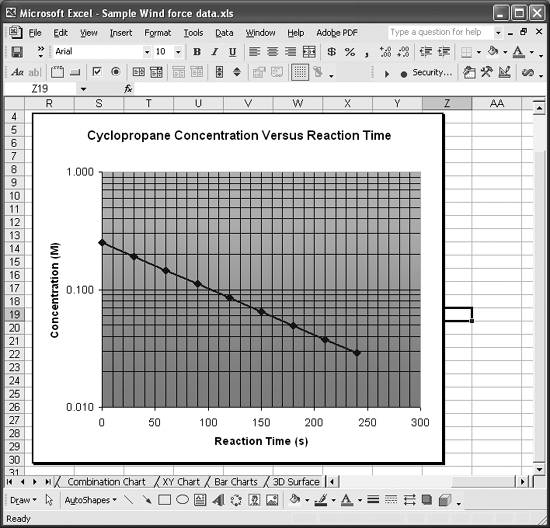# Setting Log or Semilog Scales

Problem

You'd like to use log scales instead of linear scales.

Solution

Select the axis you'd like to set to log scale and open the Format Axis dialog, as described in Recipe 4.4. Go to the Scale tab and check the Logarithmic Scale option (see Figure 4-11).

Discussion

You can create a log chart by setting both axes to logarithmic scale, or you can create a semilog chart by setting only one axis to logarithmic scale.

Consider the data shown in Figure 4-12 as an example. This data represents the reduction in concentration of cyclopropane as a function of reaction time (the cyclopropane gets converted to propane gas).

Figure 4-12. Reaction time plotThis plot of concentration versus reaction time shows the characteristic logarithmic form of so-called first-order reactions. Changing the vertical axis to logarithmic scale as described earlier results in the chart shown in Figure 4-13.

Notice that the plotted line is now linear. Plotting the data in this form facilitates estimating the rate constant for such a reaction, since the slope of the line can be determined by using the simple equation for a straight line.

Figure 4-13. Reaction time plotted on semilog chartSee Chapter 8 to learn how to perform least-squares curve fitting in Excel.Excel Scientific and Engineering Cookbook (Cookbooks (OReilly))
ISBN: 0596008791
EAN: 2147483647
Year: N/A
Pages: 206
Authors: David M Bourg